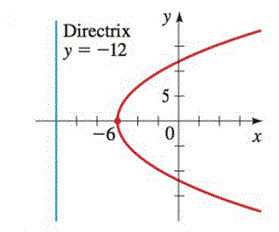Chapter 12.4, Problem 30E### Algebra and Trigonometry (MindTap ...

4th Edition
James Stewart + 2 others
ISBN: 9781305071742

#### Solutions

Chapter
Section### Algebra and Trigonometry (MindTap ...

4th Edition
James Stewart + 2 others
ISBN: 9781305071742
Textbook Problem

# 29-34 ■Finding the Equation of Shifted Conic Find an equation for the conic whose graph is shown.To determine

To find:

An equation for the conic whose graph is shown.

Explanation

Given:

The graph

Approach:

From given graph one can find out vertex and the directrix. Then the standard equation of shifted parabola facing positive x-axis can be used

(yk)2=4p(xh)

Calculation:

We can find the vertex (h,k) from the graph and its value is (6,0), so h=6 and k=0.

Now by putting the value of (h,k) in the standard equation of shifted parabola facing positive x-axis.

(y0)2=4p(x(6))

y2=4p(x+6)

In the graph equation of directrix y=12 is also given

### Still sussing out bartleby?

Check out a sample textbook solution.

See a sample solution

#### The Solution to Your Study Problems

Bartleby provides explanations to thousands of textbook problems written by our experts, many with advanced degrees!

Get Started